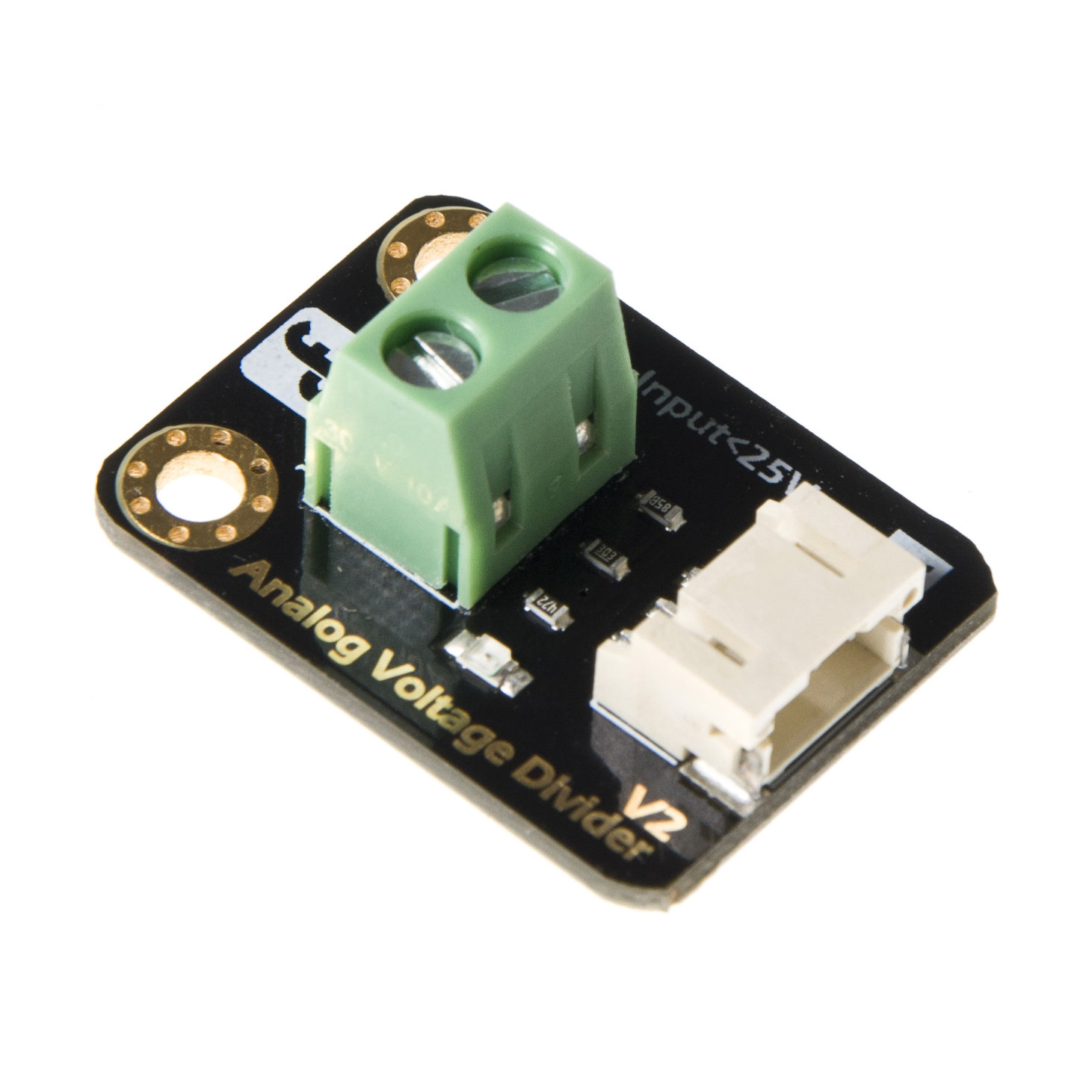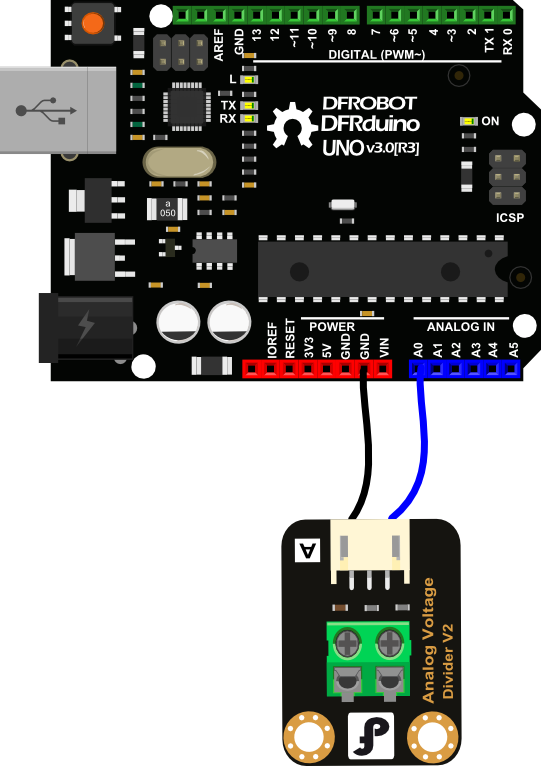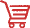# Gravity: Analog Voltage Divider V2 WIKI-DFRobot## Introduction

Analog Voltage Divider (SKU: DFR0051) The Voltage Divider can detect the supply voltage up to 25V. The DFRobot Voltage Divider module is based on resistor divider principle. The voltage detection module allows the input voltage to reduce 5 times. As the Arduino analog input voltage is up to 5V, so voltage detection module's input voltage can not be greater than the 5Vx5 = 25V.

## Specification

• Interface: Analog
• Input voltage (DC): Maximum 25V, Minimum 0.0245V
• Detects the supply voltage up to 25V
• Size: 22x30mm

### Connection Diagram### Sample Code

``````void setup()
{
Serial.begin(9600);
}
void loop()
{
int val;
float temp;
val=analogRead(0);//This divider module will divide the measured voltage by 5, the maximum voltage it can measure is 25V.
temp=val/40.92; //
val=(int)temp;//
Serial.println(val);
delay(100);
}``````

## More DocumentsGet Analog Voltage Divider V2 from DFRobot Store or DFRobot Distributor.

Turn to the Top